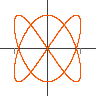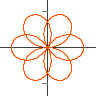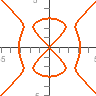Controls Java Version

This program draws the result of applying a complex function to a circle radius $r$ in the complex plane, that is all points of the form $z=r e^{i \phi}$. For a polynomial of degree $d$ and large values of $r$ this will produce a curve which loops $d$ times round the origin. For small values of $r$ when the constant and linear terms dominate it will be a small loop winding once round the constant term.

Jep is a mathematical equation parsing and evaluation library written in java. It features fast evaluation, a configurable architecture allowing custom syntax, support for BigDecimal, complex numbers and vectors It is developed by Singular Systems

GWT-Jep is a port of Jep to run in javascript and has all the features of Jep apart from a small set of changes necessary for javascript compatibility. It is compiled from Java using the Google Web Toolkit. It is available as a seperate package from SingSurf.

Example equations:

• z^2 + (bx + i by) z + cx + i cy

## Jep plotting appsGraphs
y=a x^2+b x+c
JavaScript Version
Java VersionParametric
x=cos(t), y=sin(t)
JavaScript Version
Java VersionPolar
r = cos(3 th/2)
JavaScript Version
Java VersionComplex
(z+a)/(z+b)
JavaScript VersionImplicit
x^2+y^2=1
JavaScript Version
Java Version
Msqli defined